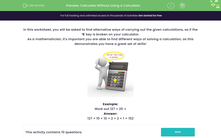# Calculate Without Using a Calculator

In this worksheet, students will be asked to find alternative ways to solve a calculation rather than using the most obvious approach. The student will be tested on addition, subtraction, multiplication and division.Key stage:  KS 2

Curriculum topic:   Number: Addition, Subtraction, Multiplication and Division

Curriculum subtopic:   Solve Multi-Step Add/Subtract Problems

Popular topics:   Subtraction worksheets, Addition worksheets

Difficulty level:#### Worksheet Overview

In this activity, you will be asked to find alternative ways of carrying out the given calculations, as if the '5' key is broken on your calculator.

As a mathematician, it's important you are able to find different ways of solving a calculation, as this demonstrates you have a great set of skills!Example:

Work out 127 + 25 =

127 + 10 + 10 + 2 + 2 + 1 = 152

Let's try some questions.

### What is EdPlace?

We're your National Curriculum aligned online education content provider helping each child succeed in English, maths and science from year 1 to GCSE. With an EdPlace account you’ll be able to track and measure progress, helping each child achieve their best. We build confidence and attainment by personalising each child’s learning at a level that suits them.

Get started